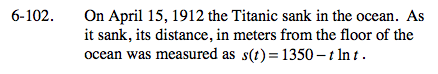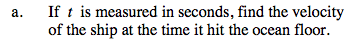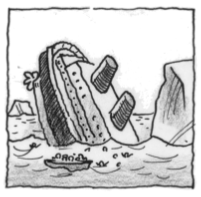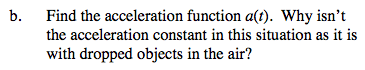### Home > CALC > Chapter 6 > Lesson 6.3.3 > Problem6-102

6-102.
1. On April 15, 1912 the Titanic sank in the ocean. As it sank, its distance, in meters from the floor of the ocean was measured as s(t) = 1350 − t ln t. Homework Help ✎

1. If t is measured in seconds, find the velocity of the ship at the time it hit the ocean floor.

2. Find the acceleration function a(t). Why isn't the acceleration constant in this situation as it is with dropped objects in the air?Use the position function to find the time the Titanic hit the ocean floor.

Evaluate the velocity function at that time.Acceleration is known to be constant on Earth: −32 ft/sec². This constant is often used in problems involving falling objects (like soccer balls). How is a sinking ship different from a falling ball?